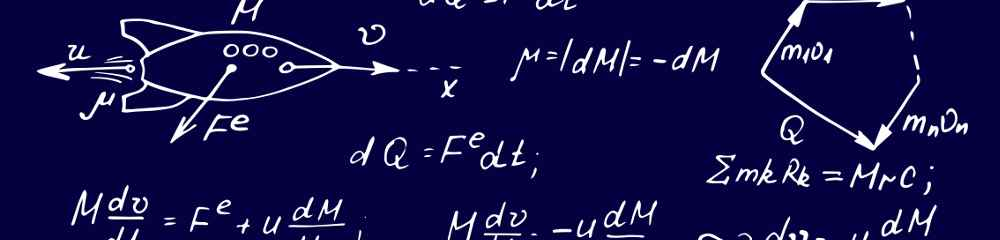# What is Modeling?

The art of creating mathematical descriptions of physical phenomena.

The Pythagoreans believed that the true nature of reality is mathematical. Though we may never know if that is so, modern scientific thought centers around the belief that mathematical models can describe reality with increasing precision. Modeling is the art of creating mathematical descriptions of physical phenomena.

These mathematical models usually take one of two forms:

Automata are made up of a description of state and rules to follow for the state to evolve. The evolution of the state describes and predicts the behavior of the modeled phenomenon.

Equations are mathematical descriptions of rules that some physical phenomenon is expected to follow.

The two are not exclusive — the “rules of evolution” of an automaton are described mathematically by the the very object created to signify a rule — the equation! It may thus be said that all mathematical models are made up of equations. The art of modeling thus boils down to creating a set of equations that will describe a physical phenomenon of interest to some degree of accuracy.

At Noumenon Multiphysics, every project starts with modeling. Various kinds of models, including ordinary and partial differential equations, integro-differential equations, homogenized models, discrete event models, queuing theoretical models, graph theoretical models, Markov chain models, etc. have been employed by us to model various observable phenomena.

WHAT IS SIMULATION. Simulation is the art of using mathematical models to approximate reality inside a computer. When used correctly, it proves to be an extremely efficient and beneficial tool for the industry. Read more…

MODELING & THE INDUSTRY. Industry depends on physical phenomena. This much is easy to assert. Two questions arise — first, are mathematical models of such phenomena important, and, second, don't they already exist in textbooks anyway? Read more…

DATA DRIVEN VS. PHYSICS AWARE MODELING. The most basic or “data driven” form of modeling involves performing a lot of experiments, and finding a mathematical relation that best explains all the data that comes out. Read more…

EXAMPLE PROJECTS

Each project at Noumenon Multiphysics has an aspect of modeling to it. Following are some projects showcasing a strong modeling component:

NOUMENON MULTIPHYSICS PROVIDES MULTI-DISCIPLINARY MODELING AND SIMULATION SERVICES TO THE ENGINEERING INDUSTRY